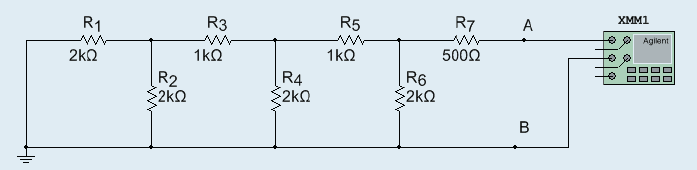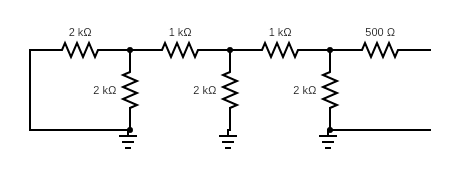# Calculating the Thevenin's Resistance of a Breadboarded Circuit

• Samama Fahim
To expand on what f95toli said, the concept of ground can vary depending on the type of circuit you are working with. In electronic circuits, ground is often used as a reference point for voltage measurements. In electrical power systems, ground is used for safety purposes and to provide a return path for current. In both cases, ground is not a physical component but rather a reference point that helps us analyze and understand the circuit.Some good resources to learn more about ground and its applications are:1. "Grounds for Grounding: A Circuit-to-System Handbook" by Elya B. Joffe and Kai-Sang Lock2. "Grounding and Shielding Techniques in Instrumentation" by Ralph Morrison3.

#### Samama FahimHow would we calculate the Thevenin's resistance for the above circuit? The right answer for this circuit is 1.5 kOhm.

$$R_{567} = R_7 + (R_5 \parallel R_6) = .5 + .67 = 1.2 k\Omega$$
$$R_{34567} = R_3 + (R_4 \parallel R_{567}) = 1 + .75 = 1.75 k\Omega$$
$$R_{TH} = R_1 + (R_2 \parallel R_{34567}) = 2 + .93 = 2.93 k\Omega,$$

which is not the right answer. Where am I making the mistake?

Last edited:
R5 and R6 are not in parallel.
Hint: start at the other end...

PS Is this homework? If so it is in the wrong sub-forum

•berkeman
(1) Which sub-forum should I rather post it in?

(2) Starting at the other end:
$$R_{123} = R_1 + (R_2 \parallel R_3) = 2 + \frac{2}{3} = \frac{8}{3}\ \text{kOhm}$$
$$R_{12345} = R_{123} + (R_4 \parallel R_5) = \frac{8}{3} + \frac{2}{3} = \frac{10}{3}\ \text{kOhm}$$
$$R_{TH} = R_{12345} + (R_6 \parallel R_7) = \frac{10}{3} + \frac{2}{5} = \frac{56}{15}\ \text{kOhm} \neq 1.5\ \text{kOhm}$$

(3) What's the key to determining whether two resistors are in parallel in a circuit at least not simple like the one above?

f95toli said:
PS Is this homework? If so it is in the wrong sub-forum
I moved it to the schoolwork forums.

Samama Fahim said:
What's the key to determining whether two resistors are in parallel in a circuit at least not simple like the one above?

It can be tricky sometimes.
In this case I believe the "key" is to realize that all the nodes at the bottom (which connects R2,R4 and R6) are grounded and at the same potential
It is therefore perfectly fine to re-draw the circuit and replace the sole ground symbol a the bottom left corner with individual ground connections for each resistor (R2, R4 and R6), once you've done this you can "cut" the leads between R2 and R4, R4 and R6 without changing the circuit in any way.

Try this. It should make it easier to see which resistors are in parallel.

Also,don't try to do too much as once. "Replace" two resistor at a time with a new resistor. Start in the left hand corner.

Edit: replaced some confusing language

Last edited:It looks like R1 and R2 are in parallel, and R12 is in series with R3. And R4 and R5 are in parallel and R3 is in series with R45. Then R6 and R7 are in parallel. Is that right?

You are on the right track.

You do not need ground unless you are computing currents and voltages.
Slide the ground symbol along to point B.
Recognise the pattern. The circuit you have is called an R:2R ladder.

Notice that...
If two identical value resistors are in parallel, the equivalent is half the value of one resistor.
If two resistors are in series, the equivalent is the sum of the two.

You should be able to progressively reduce the circuit to the thevenin equivalent, from left to right, without writing an equation.

•Merlin3189 and Joshy
Samama Fahim said:
View attachment 267894

It looks like R1 and R2 are in parallel, and R12 is in series with R3. And R4 and R5 are in parallel and R3 is in series with R45. Then R6 and R7 are in parallel. Is that right?

Yes, that is what I meant.

I should point out that my description above wasn't very good. Current CAN of course flow to ground in the lower lead (meaning current is strictly speaking flowing between the bottom nodes, although they are at the same potential).
I was trying to explain why re-drawing the circuit the way you did is fine, but I should have been more careful in my wording

Baluncore's suggestion of simply "sliding" the ground symbol to B would have been a better suggestion.

•berkeman
$$R_{12} = R_1 \parallel R_2 = 1$$
$$R_{123} = R_{12} + R_3 = 2$$
$$R_{1234} = R_{123} \parallel R_4 = 1$$
$$R_{12345} = R_{1234} + R_5 = 2$$
$$R_{123456} = R_{12345} \parallel R_6 = 1$$
$$R_{\text{TH}} = R_{123456} + R_7 = 1.5$$

@f95toli @Baluncore thanks.

Could any of you suggest me a book or some article that would discuss concept like the ground and where its use is appropriate?

Samama Fahim said:
that would discuss concept like the ground
It is not some additional component in a circuit. Ground is just a node which is "assigned" a potential of 0V. All other node voltages are w.r.t the ground node. You can choose any node as the ground node, just be consistent with directions and polarities.

•Merlin3189